# Quiz 8: Receivables

Business

Classification of Receivables In any organization receivables are generally from the sales in the normal course of the business. These generally include accounts receivables or the notes receivables. The term receivables generally include any claim in terms of money against any entity. Apart from the two types of receivables mentioned above, there exist one more type of receivables which is called other receivables and it may include interest or tax receivable. a.Accounts receivables turnover is a measure that is useful for evaluating the receivables movement or say how the receivables are converted into cash. The frequency into cash from receivables can be measured with the analysis of the turnover and number of days.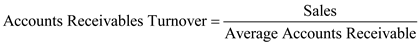With the help of formula given above accounts receivable turnover can be calculated In the present case, the accounts receivables turnover for A.com, Inc. and BB Inc can be calculated using the above mentioned formula. In case of company A.com the sales are \$88,988 and the calculation of average accounts receivables is given below:Hence average account receivable is \$5,189.5 which will further be used while calculating account receivables turnover. Given below is the calculation of accounts receivable turnover derived by using the values calculated above and the one given in questions:Thus, the accounts receivable turnover in case of company A comes out to be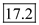The accounts receivables turnover for A.com, Inc. and BB Inc can be calculated using the above mentioned formula. In case of BB Inc. the sales are \$40,339 and the calculation of average accounts receivables is given below:Hence average account receivable is \$1,294 which will further be used while calculating account receivables turnover. Given below is the calculation of accounts receivable turnover derived by using the values calculated above and the one given in questions:Thus, the accounts receivable turnover in case of company A comes out to be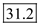b.Number of day's sales in receivable is a measure that is useful for evaluating the receivables movement or say how the receivables are converted into cash. The frequency into cash from receivables can be measured with the analysis of the turnover and number of days.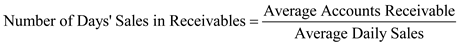With the help of formula given above Number of days' sales in receivable can be calculated In the case of A.com the number of days' sales in receivables for A.com is computed using the equation mentioned above. In the present case the average accounts receivables is \$5,189.5 and the calculation of average daily sales is given below:Hence the average account receivable is \$243.8 which will be used in the calculation of number of days in receivable. It was assumed that the number of days in a year is 365.Given below is the calculation of number of day's sales in receivable:Thus, the number of day's sales in receivables in case of A.com comes out to be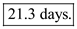In the case of BB Inc the number of days' sales in receivables is computed using the equation mentioned above. In the present case the average accounts receivables as computed above is \$1,294 and the calculation of average daily sales is given below:Hence the average daily sales is \$110.52 which will be used in the calculation of number of day's sales in receivables. It was assumed that the number of days in a year is 365.Given below is the calculation of number of day's sales in receivable:Thus, the number of day's sales in receivables in case of BB Inc comes out to be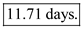c.From calculation done in part A and B, the data of A.com and BB Inc. regarding the Number of day's sales in receivable and account receivable turnover can be summarized in the table given below: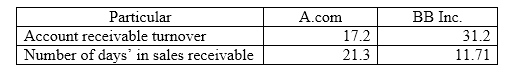The number of Days' Sales in receivables for BB Inc. is higher as compared to A.com Now in A.com the turnover of receivables are lower as compared to BB Inc. These could be because of the longer credit given to customers and as a result it takes more time to convert into cash. d.This difference explains that the credit terms policy of A.com needs to be changed to that level where it can quickly converts the receivables into cash. It also shows that BB Inc. is more efficient in collecting its accounts receivable than A.com

Direct Write-off Method Direct Write-off Method is used by those who have higher cash sales. Under Direct Write-off Method, the customer account is not credited till the time the amount receivables from that customer's account determined to be uncollectible. In some cases the amount that already determined as uncollectible and has been write-off may be collected later then in that case the collected amount needs to be reversed and shown as income. Uncollectible Receivables Some companies may shift their receivables to other by accepting only cash or credit card or via selling receivables to factoring services. Regardless of the company policies some receivables will be uncollectible and we have to record that amount as an operating expenses as a Bad Debt Expenses. There are so many reasons for consideration of the symptoms of Bad Debt such as no response, past due, files for bankruptcy, closes its business. In this case, the journalization of the transactions using the direct write-off method of accounting for uncollectible receivables are as under will be made as shown under. Amount of bad debt can be ascertain as follow:On the account receivable cash is received and the balance is recognized as bad debt.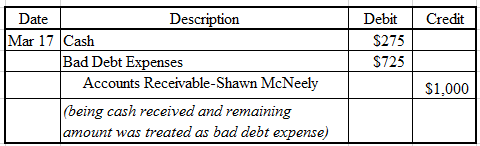\$275 is received from receivable account of Shawn McNeely in cash and the balance of \$725 has been written off from his account by debiting the two accounts and the receivable account is credited with the amount of \$1,000. The reinstatement of account takes place by passing a reverse entry of the entry which account was written off.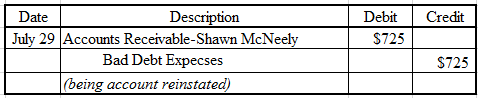Being the account receivable of Shawn McNeely reinstated which was written off earlier. The same is done by debiting the receivable account and crediting the bad expenses account. Cash against the account receivable is received that reduces the outstanding balance of account receivable.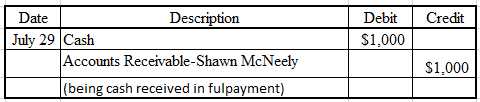Being the balance amount of receivable account amounting to \$1,000 is received in cash and the same is reflected by debiting cash account and crediting receivable account.

The three classifications of receivables are; accounts receivable, notes receivable, and other receivables.